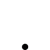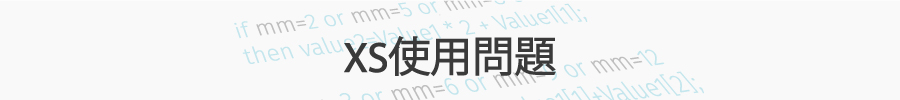如何取得進場價？

•   291
• 最後發表   大師  2018 九月 22

（策略概念如下圖）

GammaCEO 發文於   2018/09/16

value1=變數一(MA5)  value2=變數二

condition1=close 條件或算式 變數一 或and 或or 其他變數成立;

if condition1 and value2=0 then

begin

value2=open;//開盤價取得

ret=1 ;

retmsg=value2;

end else
begin
value2=0;
end;

-------------------------------------------------------------------------------

If open<MA5 and close>open and close>MA5
then ret=1;

If GetQuote("成交") > "X" * 1.1
then ret=1;
------------------------------------------------------------------------------

XQ小幫手 發文於   2018/09/18

Hi 大師，

GammaCEO 發文於   2018/09/18

首先，XS進場出場是不同腳本分開運作但同步執行的；

if value2=0 then ←假設要儲存的變數值為value2

begin

if condition1  then ←進場腳本觸發

begin

value2=open; ←進場價SAVE

end;

end else

begin

if value2>0 and close>= value2*1.1 or close<=value2/1.1 then ←出場條件成立

begin

ret=1;

value2=0; ←觸發後變數歸零

ertmsg="自訂";

end;

if  value2>1 and CurrentTime >= XXXXXX ←強制出場時間

then begin

ret=1;

value2=0;←一樣要歸零

RetMsg = "TIME UP強制平倉";

end;
end;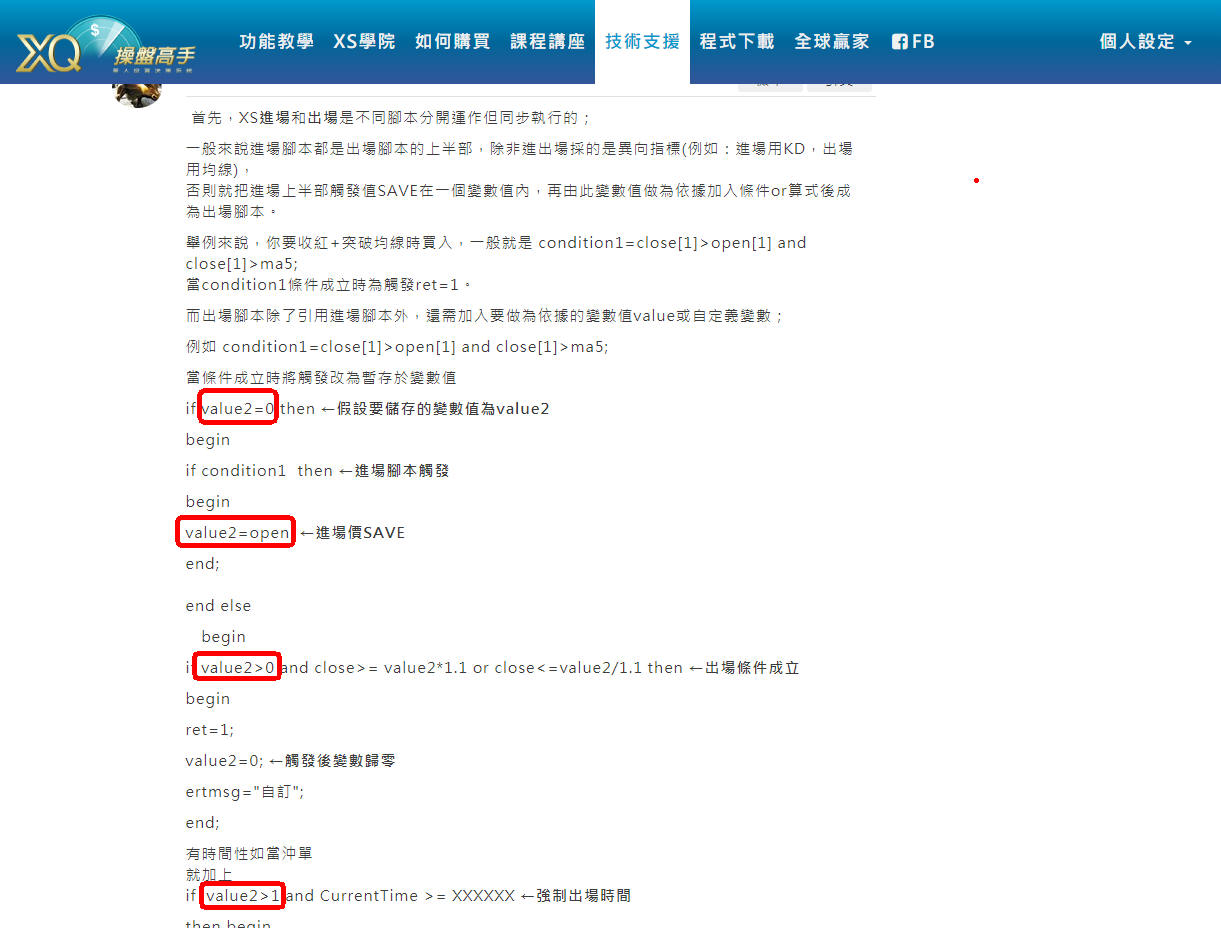GammaCEO 發文於   2018/09/22

value2是假設變數，用來記錄進場條件成立當下的價格；

value2=0 就是條件未成立，也就是未進場，大於0與大於1是同意思，表示條件已成立過，被使用了，多單或空單進場了。

value2不管等於收盤、開盤、最高或最低都會大於0，而出場判斷是建立在已進場狀況下才符合邏輯，所以須給XScript一個假設值判斷目前進場策略觸發過沒。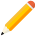發表新主題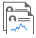我的發文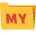我的收藏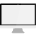分類

Close